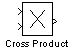# Cross Product

Cross product of two 3-D vectors

• Library:
• Simulink 3D Animation / Utilities

•## Description

Return the cross product–or vector product–of two 3-by-1 vectors. Each input is a vector of the form ${a}_{1}\stackrel{^}{i}+{a}_{2}\stackrel{^}{j}+{a}_{3}\stackrel{^}{k}$ where i, j, and k are unit vectors parallel to the x, y, and z coordinate axes. The output vector $\stackrel{\to }{y}=\stackrel{\to }{a}×\stackrel{\to }{b}$ is a 3 element vector orthogonal to the input vectors $\stackrel{\to }{a}$ and $\stackrel{\to }{b}$

## Ports

### Input

expand all

Input vector $\stackrel{\to }{a}$, where the elements represent the magnitude of the vector parallel to the x, y, and z coordinate axes.

Data Types: `double`

Input vector $\stackrel{\to }{b}$, where the elements represent the magnitude of the vector parallel to the x, y, and z coordinate axes.

Data Types: `double`

### Output

expand all

Output vector $\stackrel{\to }{y}=\stackrel{\to }{a}×\stackrel{\to }{b}$, which is orthogonal to $\stackrel{\to }{a}$ and $\stackrel{\to }{b}$

Data Types: `double`

## Extended Capabilities

### Topics

Introduced in R2006a